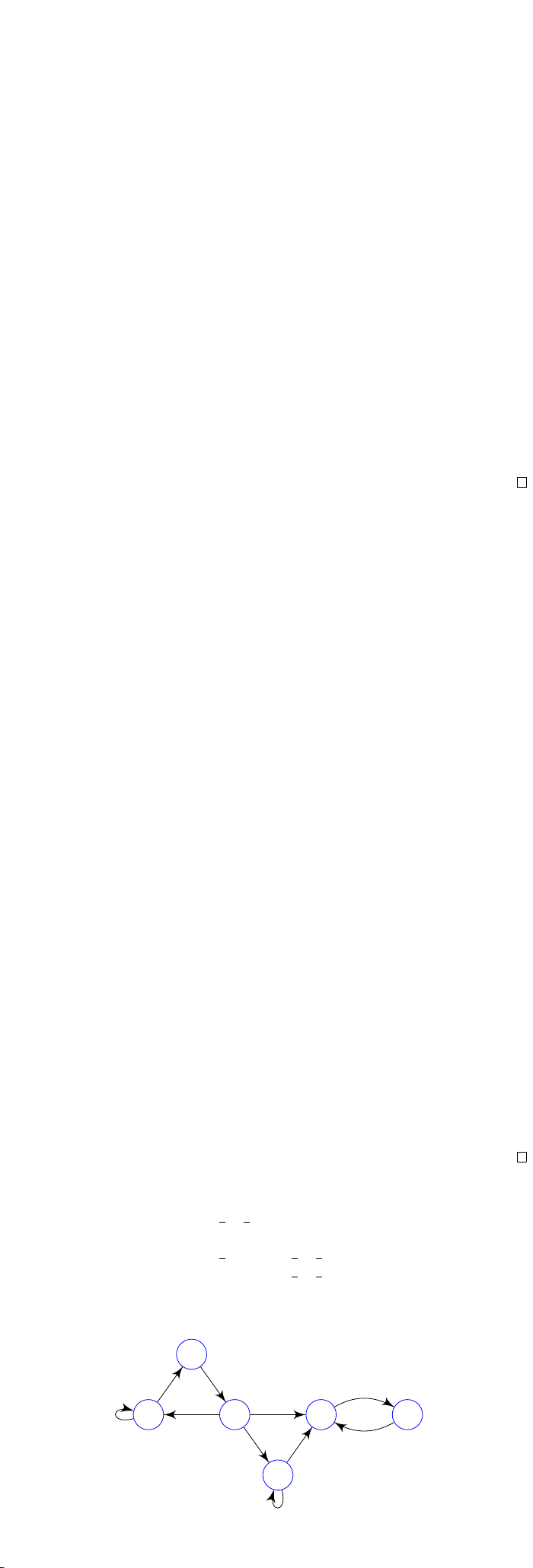2Classification of chains and states

IB Markov Chains2.1 Communicating classes
Suppose we have a Markov chain
X
over some state space
S
. While we would
usually expect the different states in
S
to be mutually interacting, it is possible
that we have a state
i S
that can never be reached, or we might get stuck in
some state
j S
and can never leave. These are usually less interesting. Hence
we would like to rule out these scenarios, and focus on what we call irreducible
chains, where we can freely move between different states.
Definition (Leading to and communicate). Suppose we have two states
i, j S
.
We write
i j
(
i
j
) if there is some
n
0 such that
p
i,j
(
n
)
>
0, i.e.
it is possible for us to get from
i
to
j
(in multiple steps). Note that we allow
n = 0. So we always have i i.
We write i j if i j and j i. If i j, we say i and j communicate.
Proposition. is an equivalence relation.
Proof.
(i) Reflexive: we have i i since p
i,i
(0) = 1.
(ii) Symmetric: trivial by definition.
(iii)
Transitive: suppose
i j
and
j k
. Since
i j
, there is some
m >
0
such that
p
i,j
(
m
)
>
0. Since
j k
, there is some
n
such that
p
j,k
(
n
)
>
0.
Then p
i,k
(m + n) =
P
r
p
i,r
(m)p
rk
(n) p
i,j
(m)p
j,k
(n) > 0. So i k.
Similarly, if
j i
and
k j
, then
k i
. So
i j
and
j k
implies
that i k.
So we have an equivalence relation, and we know what to do with equivalence
relations. We form equivalence classes!
Definition (Communicating classes). The equivalence classes of
are commu-
nicating classes.
We have to be careful with these communicating classes. Different commu-
nicating classes are not completely isolated. Within a communicating class
A
,
of course we can move between any two vertices. However, it is also possible
that we can escape from a class
A
to a different class
B
. It is just that after
going to
B
A
. From
B
, we might be able to get to
another space
C
. We can jump around all the time, but (if there are finitely
many communicating classes) eventually we have to stop when we have visited
every class. Then we are bound to stay in that class.
Since we are eventually going to be stuck in that class anyway, often, we can
just consider this final communicating class and ignore the others. So wlog we
can assume that the chain only has one communicating class.
Definition (Irreducible chain). A Markov chain is irreducible if there is a unique
communication class.
From now on, we will mostly care about irreducible chains only.
More generally, we call a subset closed if we cannot escape from it.
Definition (Closed). A subset C S is closed if p
i,j
= 0 for all i C, j ∈ C.
Proposition. A subset C is closed iff i C, i j implies j C”.
Proof.
Assume
C
is closed. Let
i C, i j
. Since
i j
, there is some
m
such
that p
i,j
(m) > 0. Expanding the Chapman-Kolmogorov equation, we have
p
i,j
(m) =
X
i
1
,··· ,i
m1
p
i,i
1
p
i
1
,i
2
, ··· , p
i
m1
,j
> 0.
So there is some route
i, i
1
, ··· , i
m1
, j
such that
p
i,i
1
, p
i
1
,i
2
, ··· , p
i
m1
,j
>
0.
Since
p
i,i
1
>
0, we have
i
1
C
. Since
p
i
1
,i
2
>
0, we have
i
2
C
. By induction,
we get that j C.
To prove the other direction, assume that
i C, i j
implies
j C
”. So
for any i C, j ∈ C, then i → j. So in particular p
i,j
= 0.
Example. Consider S = {1, 2, 3, 4, 5, 6} with transition matrix
P =
1
2
1
2
0 0 0 0
0 0 1 0 0 0
1
3
0 0
1
3
1
3
0
0 0 0
1
2
1
2
0
0 0 0 0 0 1
0 0 0 0 1 0
1
2
3
4
5 6
We see that the communicating classes are {1, 2, 3}, {4}, {5, 6}, where {5, 6} is
closed.